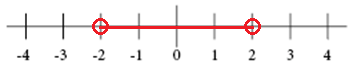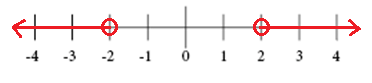# Solving absolute value equations and inequalities

The absolute number of a number a is written as

$\left | a \right |$

And represents the distance between a and 0 on a number line.

An absolute value equation is an equation that contains an absolute value expression. The equation

$\left | x \right |=a$

Has two solutions x = a and x = -a because both numbers are at the distance a from 0.

To solve an absolute value equation as

$\left | x+7 \right |=14$

You begin by making it into two separate equations and then solving them separately.

$x+7 =14$

$x+7\, {\color{green} {-\, 7}}\, =14\, {\color{green} {-\, 7}}$

$x=7$

or

$x+7 =-14$

$x+7\, {\color{green} {-\, 7}}\, =-14\, {\color{green} {-\, 7}}$

$x=-21$

An absolute value equation has no solution if the absolute value expression equals a negative number since an absolute value can never be negative.

The inequality

$\left | x \right |<2$

Represents the distance between x and 0 that is less than 2Whereas the inequality

$\left | x \right |>2$

Represents the distance between x and 0 that is greater than 2You can write an absolute value inequality as a compound inequality.